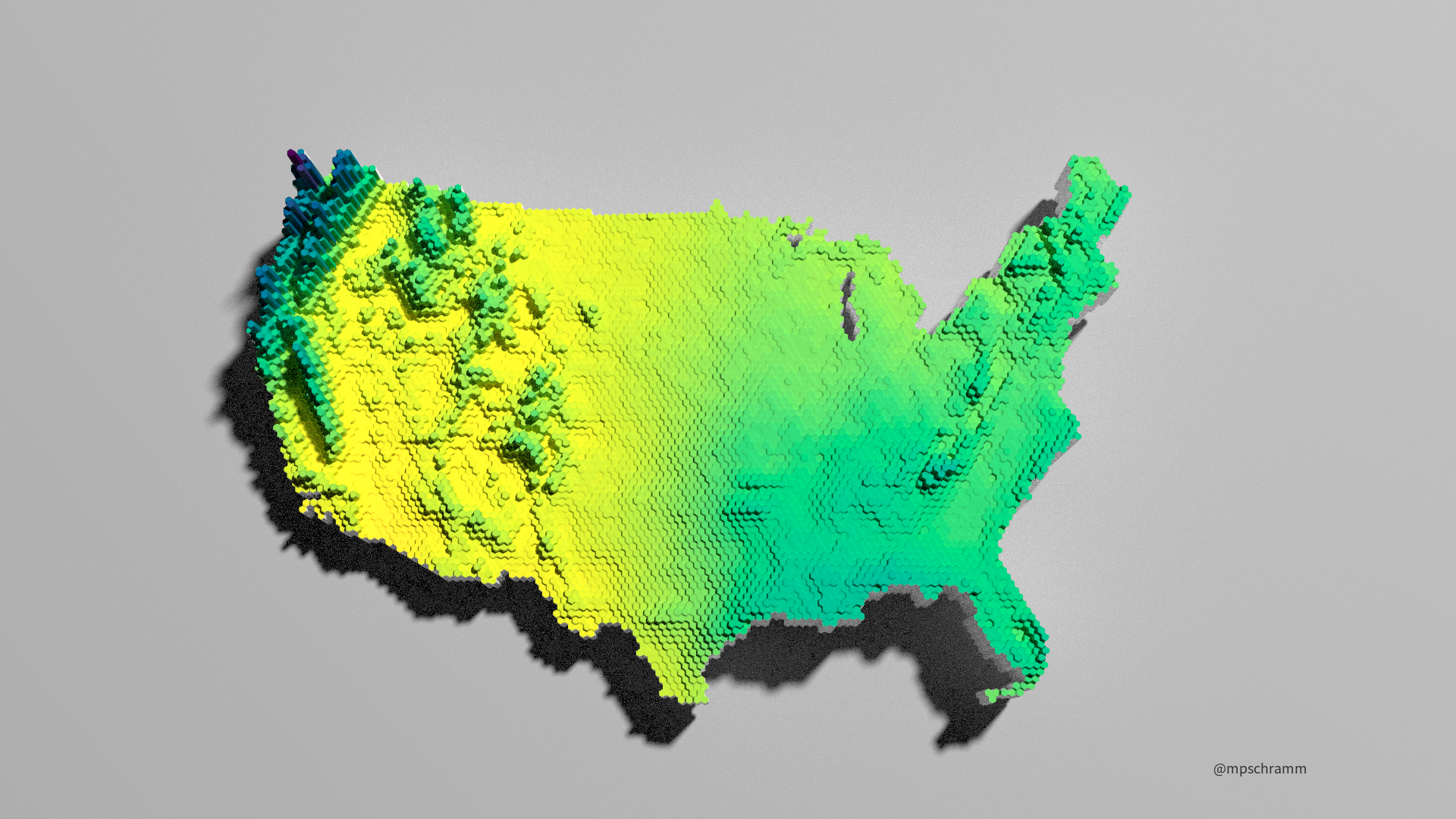I rendered some preciptiation maps of the contiguous US with rayshader.

Michael Schramm https://michaelpaulschramm.com (Texas Water Resources Institute)https://twri.tamu.edu
2021-04-15

Recently, I’ve been exploring some different gridded daily precipitation datasets and evaluating how they impact watershed models I’ve been working on. Let’s explore using the `rayshader` to make some three-dimensional maps.

First, obtain the gridded precipitation data. I will use the 30-yr mean annual precipitation from PRISM. Luckily, there is an R package so we can easily script the data download.

Second, I will smooth the data a little bit by summarizing into tiles. There is a lot of variation at the nation-wide scale by spatially-binning and averaging the data, the map is just a little more aesthetically pleasing to my eye. This can be done a bunch of different ways. Here, I will use the `sf` and `terra` packages to create and populate the hexagons with mean annual precipitation data.

Third, I will render a three-dimensional map with `rayshader`. So, load the necessary packages before we start. I’m using the development version of `rayshader` and `rayrender` because of some massive speed improvements, I recommend installing if you can (`remotes::install_github(tylermorganwall/rayshader)`; `remotes::install_github(tylermorganwall/rayrender)`). `statesRcontiguous` is only on Github, so install as follows: `remotes::install_github(charliejhadley/statesRcontiguous)`.

``````library(tidyverse)
library(rayshader) ## using v0.24.6 from Github
library(terra)
library(sf)
``````

The `prism` package downloads the gridded precipitation datasets we request, then stores the path so we can read with the `rast` function. I’m downloading the 4km resolution data, if you were doing this at the state or local level, consider downloading the 800m dataset for better resolution.

``````## PRISM data
tmpdir <- tempdir()
prism_set_dl_dir(tmpdir)
prism <- get_prism_normals("ppt", "4km", annual = TRUE, keepZip = FALSE)
``````
``````
|
|                                                            |   0%
|
|============================================================| 100%``````
``````prism_rast <- prism_archive_subset("ppt", "annual normals", resolution = "4km")
prism_rast <- pd_to_file(prism_rast)
prism_rast <- rast(prism_rast)
prism_rast <- project(prism_rast, "+proj=laea +lat_0=45 +lon_0=-100 +x_0=0 +y_0=0 +a=6370997 +b=6370997 +units=m +no_defs ")
``````

## Tile rasters

As I mentioned, there are various ways to make the tiles.[@cstats1](https://twitter.com/cstats1) posted an efficient workflow using the `summary_stat` function in `ggplot`. I wanted to project the data and utilize the new `terra` package to summarize the data. It take a bit longer, but I like the results.

Since several people have asked and I have no idea when I will actually get around to writing this process up, here are the steps to making elevation tiles in #rayshader sans pulling in the shapefiles and elevation data directly from #rstats using the Monterey Bay elevation file pic.twitter.com/0aeXnCEIk0

— newishtodc (@cstats1) February 22, 2021
``````## create and extent polygon
prism_ext <- as.polygons(ext(prism_rast), crs=crs(prism_rast))
prism_ext <- st_as_sf(prism_ext)

## create a hexagon grid in the extent polygon
hexagons <- st_make_grid(prism_ext,
n = c(150,150),
square = FALSE,
crs = crs(prism_ext))

## convert from sf back to terra,
## we lose the crs def along the way
## so set the crs again
hexagons <- vect(hexagons)
crs(hexagons) <- crs(prism_rast)

## calculate the mean raster values in each polygon
prism_summary <- extract(x = prism_rast,
y = hexagons,
fun = mean,
na.rm = TRUE)

## extract returns a matrix, need to get the data back
## into hexagons
values(hexagons) <- data.frame(ID = 1:nrow(hexagons))
hexagons <- merge(hexagons, data.frame(prism_summary))
values(hexagons) <- data.frame(prism_summary)

## what does it look like?
plot(hexagons, "PRISM_ppt_30yr_normal_4kmM2_annual_bil")
``````Time to make the computer go bananas. First, convert `hexagons` to `sf` and plot with `ggplot2`. Then we can easily generate a rayshaded plot. If you want to forgo, `ggplot2`, convert to a raster and run through `rayshader`. I usually render a low res version first to make sure I like the colors, scaling, etc. These can take quite some time to render. Play with the settings to get what you like and share on Twitter with #rayshader.

ggplot2

``````## convert to sf and features with NA values
hexagons <- st_as_sf(hexagons) %>%
filter(!is.na(PRISM_ppt_30yr_normal_4kmM2_annual_bil))

## make your ggplot, customize as needed
ggplot(hexagons) +
geom_sf(aes(fill = PRISM_ppt_30yr_normal_4kmM2_annual_bil), color = NA) +
scale_fill_viridis_c("Annual Precipition [mm]", direction = -1) +
labs(x = "Longitude", y = "Latitude", caption = "@mpschramm") +
theme(text = element_text(family = "Source Sans Pro"),
legend.position = "bottom",
legend.title = element_text(size = 8),
legend.text = element_text(size = 7),
legend.key.height = unit(0.25, "cm"),
panel.background = element_rect(fill = "white", color = "white"),
panel.grid = element_line(color = "grey10",
size = .1),
axis.title.x = element_text(size = 6, hjust = 0),
axis.title.y = element_text(size = 6, hjust = 0),
axis.text.x = element_text(size = 6),
axis.text.y = element_text(size = 6),
axis.ticks.x = element_blank(),
axis.ticks.y = element_blank()) -> p1

## make 3D ggplot
plot_gg(p1,
multicore = TRUE,
width = 4*1.777,
height = 4,
solidcolor = "white",
theta = 0,
phi = 80,
fov = 0,
zoom = .5,
background = "grey80",
windowsize = c(1920,1080))

## brrrrrr
render_highquality(lightdirection = 45,
lightaltitude = 60,
lightintensity = 1000,
samples = 1000, #lower this to get faster rendering
sample_method = "sobol",
parallel = TRUE,
width = 1920,
height = 1080,
ground_material = rayrender::diffuse(color = "grey40"),
clear = TRUE)
``````base/sp

``````## rasterize hexagons:
hexagons <- vect(hexagons)
crs(hexagons) <- crs(prism_rast)
hexagons <- rasterize(hexagons, prism_rast,
field = "PRISM_ppt_30yr_normal_4kmM2_annual_bil")
# convert from SpatRast to Raster to matrix
pptmat = raster_to_matrix(raster::raster(hexagons))
pptmat %>%
height_shade(texture = hcl.colors(100, palette = "viridis", rev = TRUE)) %>%
plot_3d(pptmat,
zscale = 16,
solidcolor = "white",
theta = 0,
phi = 80,
fov = 45,
zoom = .5,
background = "grey80",
windowsize = c(1920,1080))
render_highquality(lightdirection = 45,
lightaltitude = 60,
lightintensity = 900,
samples = 3000,
sample_method = "sobol",
parallel = TRUE,
width = 1920,
height = 1080,
ground_material = rayrender::diffuse(color = "grey40"),
clear = TRUE)
``````### Corrections

If you see mistakes or want to suggest changes, please create an issue on the source repository.

### Reuse

Text and figures are licensed under Creative Commons Attribution CC BY 4.0. Source code is available at https://github.com/mps9506/mschramm, unless otherwise noted. The figures that have been reused from other sources don't fall under this license and can be recognized by a note in their caption: "Figure from ...".

### Citation

`Schramm (2021, April 15). @mpschramm: Rayshading Precipitation Maps. Retrieved from https://michaelpaulschramm.com/posts/2021-04-15-rayshade-precipitation/`
```@misc{schramm2021rayshading,